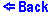Write and Plot a Function

Works much better with MS Internet Explorer 3 and higher

The above applet combination allows you plot any function and change the attributes of your graph. One can enter the function into the command line below. It must be of the form y = ..., thus y + x = 5 is not allowed. An array of functions is available, including trigonometric, logarithmic, and exponential. There is also a random number generator rand. Note that the arguments of functions must be in parentheses (ex: y=sin(x)). One can change the starting x value xmin in the command line below. The maximum x value xmax is entered just below that. The number of points numpoints determines how accurately the graph looks. Beware that large numbers may slowdown system performance. The y values of the graph are scaled automatically, so note that entering an infinite function i.e., y = tan(x) results in a huge y value. Also note that angle values for trigonometric functions are entered in radians.© Copyright 1997, Sergey Kiselev and Tanya Yanovsky-Kiselev
Last modified: June 17, 1997